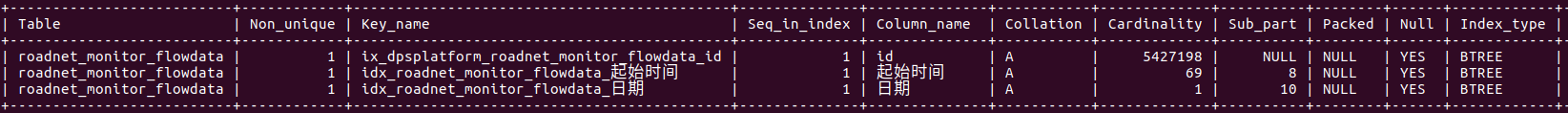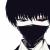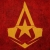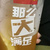我的sql语句执行非常慢，应该如何优化？

年薪达不到25W大数据工程师、拿不到Offer全额退款->>>mysql 5.7.18

```
CREATE TABLE `roadnet_monitor_flowdata` (
`id` bigint(20) DEFAULT NULL,
`交通类型` text,
`出站人数` bigint(20) DEFAULT NULL,
`日期` text,
`站点` text,
`纬度` double DEFAULT NULL,
`线路` text,
`经度` double DEFAULT NULL,
`结束时间` text,
`起始时间` text,
`进站人数` bigint(20) DEFAULT NULL,
) ENGINE=InnoDB DEFAULT CHARSET=utf8
```

```
select sum(进站人数),sum(出站人数)
where 日期= '2017-03-16'
group by 起始时间;
```01，修正表结构字段类型

2，创建索引字段0
b

00
n
CREATE TABLE `roadnet_monitor_flowdata` (
`id` bigint(20) DEFAULT NULL,
`交通类型` text,
`出站人数` bigint(20) DEFAULT NULL,
`日期` text,
`站点` text,
`纬度` double DEFAULT NULL,
`线路` text,
`经度` double DEFAULT NULL,
`结束时间` text,
`起始时间` text,
`进站人数` bigint(20) DEFAULT NULL,
KEY `idx_date` (`日期`(10)) USING HASH
) ENGINE=InnoDB DEFAULT CHARSET=utf8;

0
n
#! /usr/bin/env python
# -*- coding: utf-8 -*-
#
# gen_sql.py

import random
import time

template = '''INSERT INTO `roadnet_monitor_flowdata` VALUES(%d, "", %d, "%s", "", "", "", "", "%s", "%s", %d);''';

def random_date():
y = random.randint(2000,2017)
m = random.randint(1,12)
d = random.randint(1,30)

date = "%04d-%02d-%02d" % (y,m,d)

return date

def main():
random.seed(time.clock())
max = 5000000
for i in range(max):
id = random.randint(1,max)
num_in = random.randint(1,max/10)
num_out = random.randint(1,max/10)
date = random_date()
start = random_date()
end = random_date()

print template % (id, num_in, date, start, end, num_out);

if __name__ == "__main__":
main()

python gen_sql.py >i500w.sql